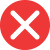52nd

Correct spelling, explanation: 52nd is the correct form since it is pronounced fifty-second – if there is number 2 at the end of a number and we want to create an ordinal one, it transforms to second and its numeral form is nd. Th is a usual suffix for ordinal numbers, but number 2 is one of the exceptions. Therefore 52th is an incorrect form and the correct one is 52nd.

Definition of 52nd:
numeral, the ordinal number between 51st and 53rd
Can you imagine today’s my grandparents’ 52nd wedding anniversary? This is absolutely wonderful.52th

Incorrect spelling, explanation: suffix -th is added to most numbers when creating an ordinal form, however, 1, 2, and 3 are the exceptions. If a number is 1, 2, or 3 or ends with one of these numbers (so is pronounced with one, two, or three at the end; like fifty-two), the ordinal suffix will be respectively -st, -nd and -rd (so pronounced with first, second or third at the end, like fifty-second). Taking this into consideration the correct form is 52nd, not 52th.52st

Incorrect spelling52rd

Incorrect spelling